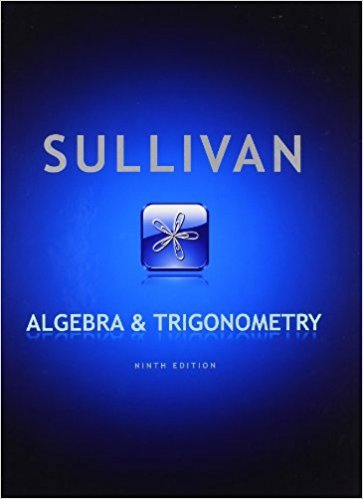×
×

# Solutions for Chapter 12.4: Algebra and Trigonometry 9th Edition## Full solutions for Algebra and Trigonometry | 9th Edition

ISBN: 9780321716569Solutions for Chapter 12.4

Solutions for Chapter 12.4
4 5 0 381 Reviews
26
5
##### ISBN: 9780321716569

Since 93 problems in chapter 12.4 have been answered, more than 61135 students have viewed full step-by-step solutions from this chapter. Chapter 12.4 includes 93 full step-by-step solutions. Algebra and Trigonometry was written by and is associated to the ISBN: 9780321716569. This expansive textbook survival guide covers the following chapters and their solutions. This textbook survival guide was created for the textbook: Algebra and Trigonometry, edition: 9.

Key Math Terms and definitions covered in this textbook
• Affine transformation

Tv = Av + Vo = linear transformation plus shift.

• Back substitution.

Upper triangular systems are solved in reverse order Xn to Xl.

• Big formula for n by n determinants.

Det(A) is a sum of n! terms. For each term: Multiply one entry from each row and column of A: rows in order 1, ... , nand column order given by a permutation P. Each of the n! P 's has a + or - sign.

• Cayley-Hamilton Theorem.

peA) = det(A - AI) has peA) = zero matrix.

• Complex conjugate

z = a - ib for any complex number z = a + ib. Then zz = Iz12.

• Distributive Law

A(B + C) = AB + AC. Add then multiply, or mUltiply then add.

• Fibonacci numbers

0,1,1,2,3,5, ... satisfy Fn = Fn-l + Fn- 2 = (A7 -A~)I()q -A2). Growth rate Al = (1 + .J5) 12 is the largest eigenvalue of the Fibonacci matrix [ } A].

• Free variable Xi.

Column i has no pivot in elimination. We can give the n - r free variables any values, then Ax = b determines the r pivot variables (if solvable!).

• Hermitian matrix A H = AT = A.

Complex analog a j i = aU of a symmetric matrix.

• Krylov subspace Kj(A, b).

The subspace spanned by b, Ab, ... , Aj-Ib. Numerical methods approximate A -I b by x j with residual b - Ax j in this subspace. A good basis for K j requires only multiplication by A at each step.

• lA-II = l/lAI and IATI = IAI.

The big formula for det(A) has a sum of n! terms, the cofactor formula uses determinants of size n - 1, volume of box = I det( A) I.

• Least squares solution X.

The vector x that minimizes the error lie 112 solves AT Ax = ATb. Then e = b - Ax is orthogonal to all columns of A.

• Length II x II.

Square root of x T x (Pythagoras in n dimensions).

• Lucas numbers

Ln = 2,J, 3, 4, ... satisfy Ln = L n- l +Ln- 2 = A1 +A~, with AI, A2 = (1 ± -/5)/2 from the Fibonacci matrix U~]' Compare Lo = 2 with Fo = O.

• Network.

A directed graph that has constants Cl, ... , Cm associated with the edges.

• Pivot columns of A.

Columns that contain pivots after row reduction. These are not combinations of earlier columns. The pivot columns are a basis for the column space.

• Pseudoinverse A+ (Moore-Penrose inverse).

The n by m matrix that "inverts" A from column space back to row space, with N(A+) = N(AT). A+ A and AA+ are the projection matrices onto the row space and column space. Rank(A +) = rank(A).

• Random matrix rand(n) or randn(n).

MATLAB creates a matrix with random entries, uniformly distributed on [0 1] for rand and standard normal distribution for randn.

• Semidefinite matrix A.

(Positive) semidefinite: all x T Ax > 0, all A > 0; A = any RT R.

• Symmetric matrix A.

The transpose is AT = A, and aU = a ji. A-I is also symmetric.

×